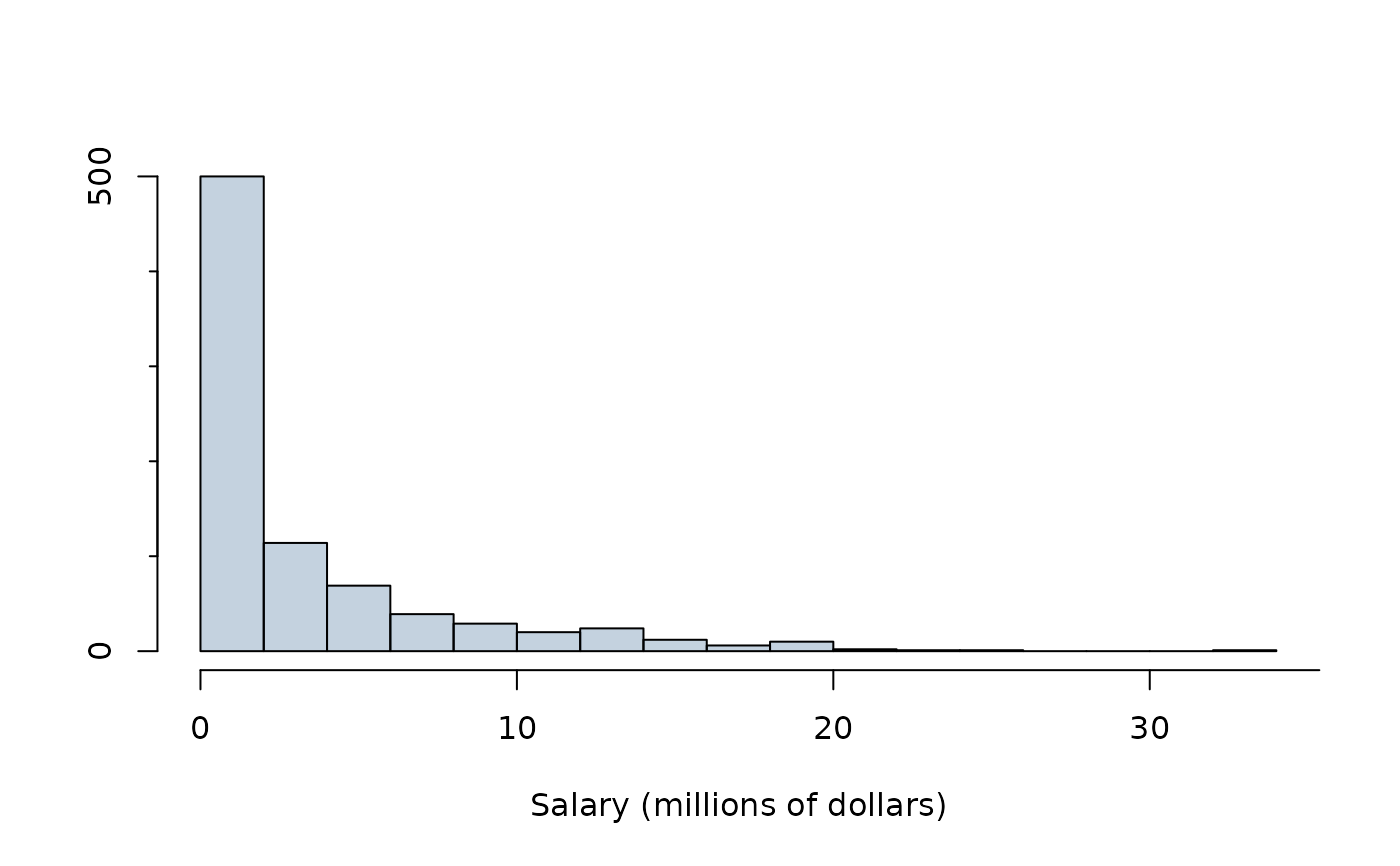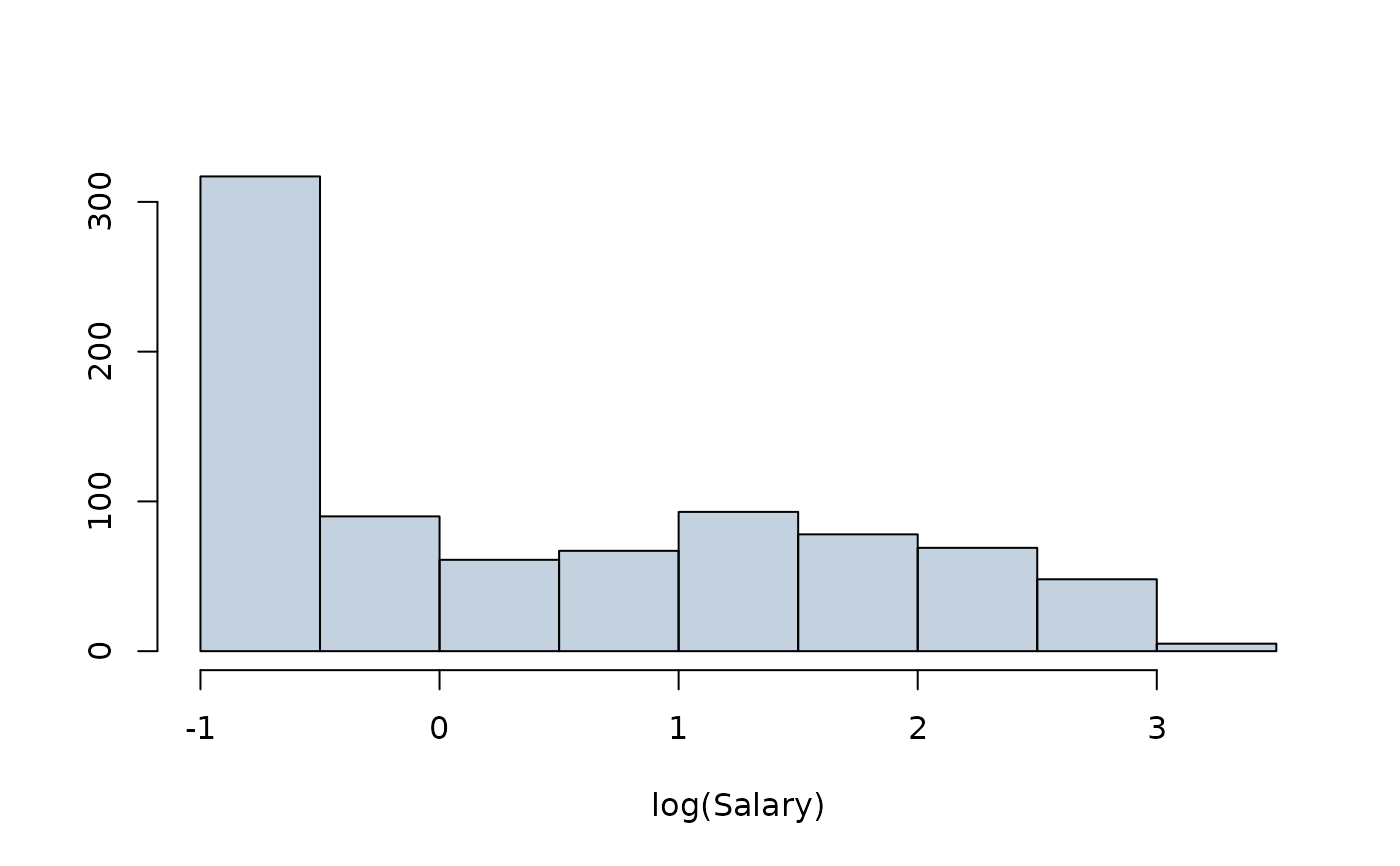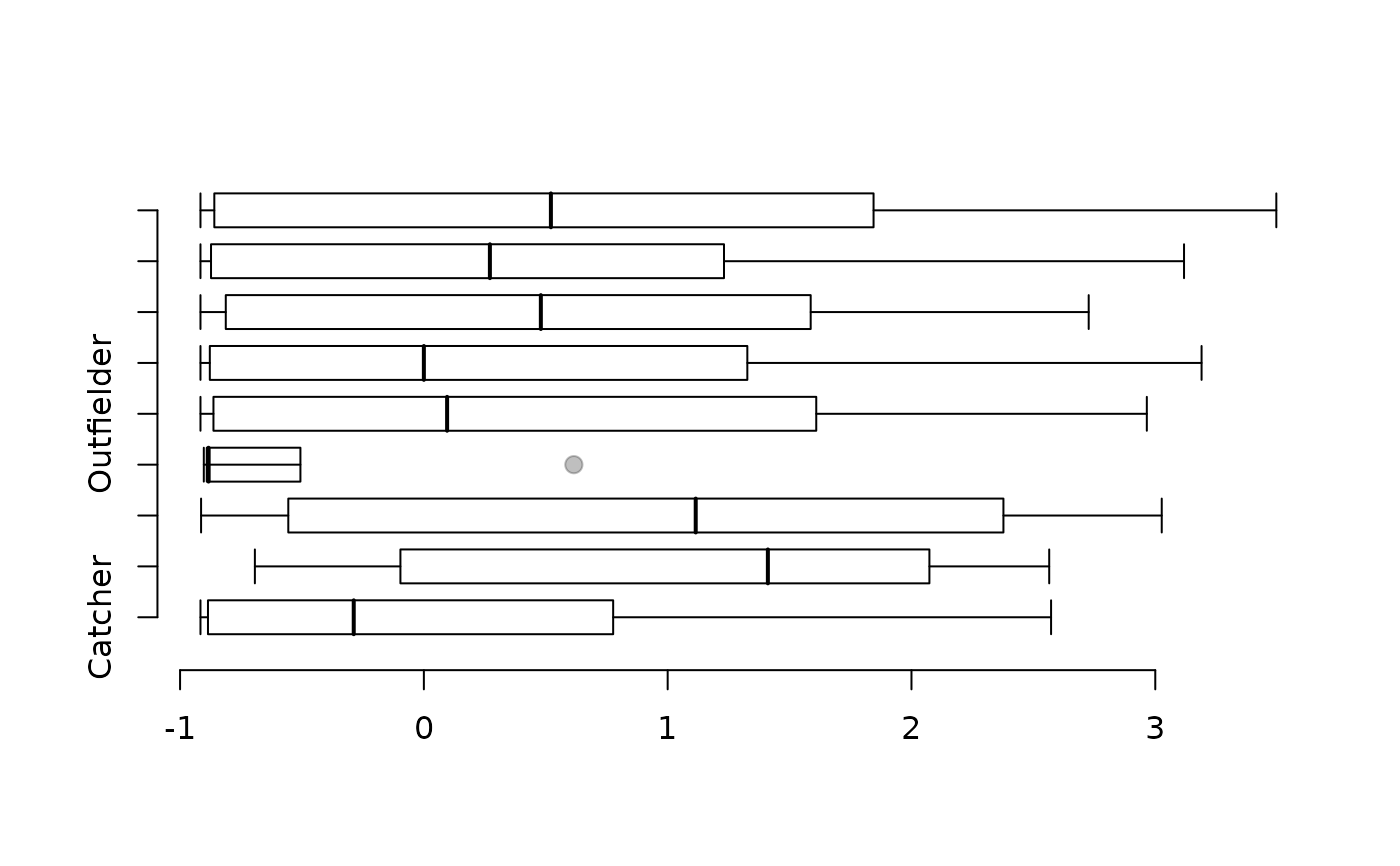Salary data for Major League Baseball players in the year 2010.

## Usage

mlb

## Format

A data frame with 828 observations on the following 4 variables.

player

Player name

team

Team

position

Field position

salary

Salary (in $1000s) ## Source https://databases.usatoday.com/mlb-salaries/, retrieved 2011-02-23. ## Examples  # _____ Basic Histogram _____ # hist(mlb$salary / 1000,
breaks = 15,
main = "", xlab = "Salary (millions of dollars)", ylab = "",
axes = FALSE,
col = "#22558844"
)
axis(1, seq(0, 40, 10))
axis(2, c(0, 500))
axis(2, seq(100, 400, 100), rep("", 4), tcl = -0.2)# _____ Histogram on Log Scale _____ #
hist(log(mlb$salary / 1000), breaks = 15, main = "", xlab = "log(Salary)", ylab = "", axes = FALSE, col = "#22558844" ) axis(1) # , seq(0, 40, 10)) axis(2, seq(0, 300, 100))# _____ Box plot of log(salary) against position _____ # boxPlot(log(mlb$salary / 1000), mlb\$position, horiz = TRUE, ylab = "")